# Two gardeners

The garden with an area of 81 square meters was divided by two gardeners in a ratio of 4: 5. How much did the second gardener get more first?

x =  9 m2

### Step-by-step explanation: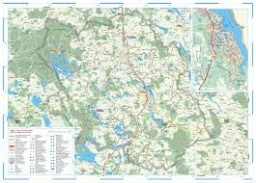Did you find an error or inaccuracy? Feel free to write us. Thank you!Tips to related online calculators
Check out our ratio calculator.

#### You need to know the following knowledge to solve this word math problem:

We encourage you to watch this tutorial video on this math problem:

## Related math problems and questions:

• Square garden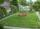On the plan with a scale of 1:1500 is drawn as a square garden with area 81 cm2. How many meters is long garden fence? Determine the actual acreage gardens.
• Extending square gardenMrs. Petrová's garden had the shape of a square with a side length of 15 m. After its enlargement by 64 m2 (square), it had the shape of a square again. How many meters has the length of each side of the garden been extended?
• 1.5 divided1.5 divided by 1 = w divided by 4
• GardenThe rectangular garden has dimensions of 27 m and 30 m. Peter and Katka split it in a ratio of 4:5. How many square meters did Katkin measure part of the garden?
• AndreAndre, Thomas, and Ivan split 88 postage stamps in a 2:5:4 ratio. How much did Thomas get?
• GardenThe area of a square garden is 2/9 of triangle garden with sides 160 m, 100 m, and 100 m. How many meters of fencing need to fence a square garden?
• Composite ratioJakub, Aneta, and Lenka divided 1342 USD in the ratio 5/2: 3/10: 1/4. How much did Lenka take?
• In theIn the national park, the ratio of the wooded area to grassland is 4: 1. The total area is 385km2. What area is wooded?
• GardensThe area of the square garden is 3/4 of the area of the triangular garden with sides of 80 m, 50 m, 50 m. How many meters of the fence do we need to fence a square garden?
• A tileA tile setter is covering 5ft by 5ft square shower wall. Each tile covers 4 5/8in by 4 5/8in square. How many rows of tile are needed to reach 5ft? How many tiles are needed to cover 5ft by 5ft square
• Divide 5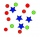Divide 288 in the following ratio 3 : 4 : 5
• MohammedMohammed grew a garden last year. His plot of land was 6 3/4 feet by 10 feet. He wants to have twice as much area and his garden this year. How much area will the new garden take up?
• Two brothersThe two brothers were to be divided according to the will of land at an area of 1ha 86a 30m2 in a ratio of 5:4. How many will everyone get?
• Gardens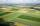I have a garden in the shape of a square with a side length of 0.02 kilometers. Neighbor has a large garden as well and one side of the garden we share. I'd bought from a neighbor garden that my area of my garden increased to 5 ares. a/ By how many acres
• Three workersThe company rewarded three workers CZK 9200, and the money was divided by the work they have done. The first worker to get twice than the second, the second three times more than the third. How much money did each worker receive?
• Three 43Three brothers inherited a cash amount of 62,000, and they divided it among themselves in the ratio of 5:4:1. How much more is the largest share than the smallest share?
• Flowerbed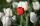Family cultivated tulips on a square flower bed of 6 meters. Later they added the square terrace with a side of 7 meters to their house. One vertex of the terrace lay exactly in the middle of a tulip bed, and one side of the terrace divided the side of th